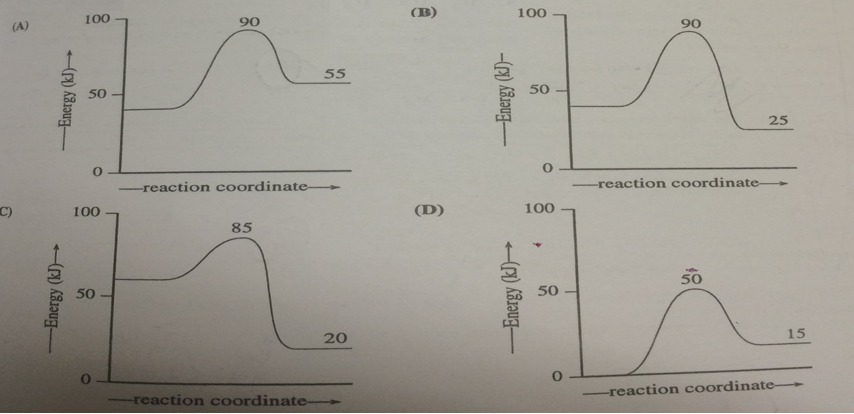Problem: Which reaction coordinate diagram represents a reaction in which the activation energy, Ea, is 50 kj.mol-1 and the ΔHrxn is -15 kj. mol-1?

96% (19 ratings)
Problem Details

Which reaction coordinate diagram represents a reaction in which the activation energy, Ea, is 50 kj.mol-1 and the ΔHrxn is -15 kj. mol-1?